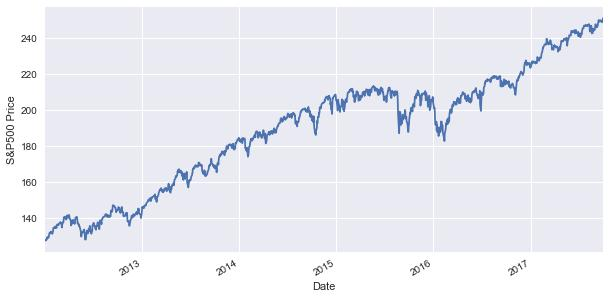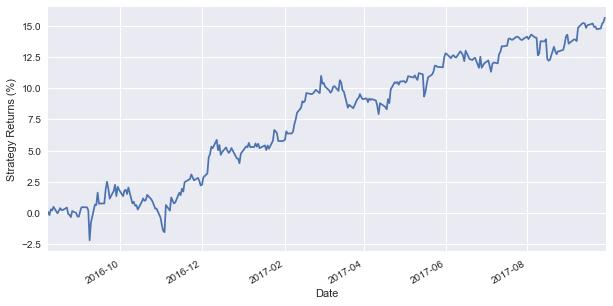# Machine Learning Classification Strategy In Python

In this blog, we will step by step implement a machine learning classification algorithm on S&P500 using Support Vector Classifier (SVC). SVCs are supervised learning classification models. A set of training data is provided to the machine learning classification algorithm, each belonging to one of the categories. For instance, the categories can be to either buy or sell a stock. The classification algorithm builds a model based on the training data and then, classifies the test data into one of the categories.

Now, let’s implement the machine learning in Python classification strategy.

### Step 1: Import the libraries

In this step, we will import the necessary libraries that will be needed to create the strategy.

# machine learning classification
from sklearn.svm import SVC
from sklearn.metrics import accuracy_score

# For data manipulation
import pandas as pd

# To plot
import matplotlib.pyplot as plt
import seaborn

### Step 2: Fetch data

After that, we will drop the missing values from the data and plot the S&P500 close price series.

import yfinance as yf
Df = Df.dropna()
Df.Close.plot(figsize=(10,5))
plt.ylabel("S&P500 Price")
plt.show()### Step 3: Determine the target variable

The target variable is that variable which the machine learning classification algorithm will predict. In this example, the target variable is whether S&P500 price will close up or close down on the next trading day.

We will first determine the actual trading signal using the following logic - if next trading day's close price is greater than today's close price then, we will buy the S&P500 index, else we will sell the S&P500 index. We will store +1 for the buy signal and -1 for the sell signal.

y = np.where(Df['Close'].shift(-1) > Df['Close'],1,-1)

### Step 4: Creation of predictors variables

The X is a dataset that holds the predictor's variables which are used to predict target variable, ‘y’. The X consists of variables such as 'Open - Close' and 'High - Low'. These can be understood as indicators based on which the algorithm will predict the option price.

Df['Open-Close'] = Df.Open - Df.Close
Df['High-Low'] = Df.High - Df.Low
X=Df[['Open-Close','High-Low']]

In the later part of the code, the machine learning classification algorithm will use the predictors and target variable in the training phase to create the model and then, predict the target variable in the test dataset.

### Step 5: Test and train dataset split

In this step, we will split data into the train dataset and the test dataset.

1. First, 80% of data is used for training and remaining data for testing
2. X_train and y_train are train dataset
3. X_test and y_test are test dataset
split_percentage = 0.8
split = int(split_percentage*len(Df))
# Train data set
X_train = X[:split]
y_train = y[:split]
# Test data set
X_test = X[split:]
y_test = y[split:]

### Step 6: Create the machine learning classificationmodel using the train dataset

We will create the machine learning in python classification model based on the train dataset. This model will be later used to predict the trading signal in the test dataset.

cls = SVC().fit(X_train, y_train)

### Step 7: The classification model accuracy

We will compute the accuracy of the classification model on the train and test dataset, by comparing the actual values of the trading signal with the predicted values of the trading signal. The function accuracy_score() will be used to calculate the accuracy.

Syntax: accuracyscore(targetactualvalue,targetpredicted_value)

1. target_actual_value: correct signal values
2. target_predicted_value: predicted signal values
accuracy_train = accuracy_score(y_train, cls.predict(X_train))
accuracy_test = accuracy_score(y_test, cls.predict(X_test))
print('\nTrain Accuracy:{: .2f}%'.format(accuracy_train*100))
print('Test Accuracy:{: .2f}%'.format(accuracy_test*100))

An accuracy of 50%+ in test data suggests that the classification model is effective.

### Step 8: Prediction

We will predict the signal (buy or sell) for the test data set, using the cls.predict() function. Then, we will compute the strategy returns based on the signal predicted by the model in the test dataset. We save it in the column 'Strategy_Return' and then, plot the cumulative strategy returns.

Df['Predicted_Signal'] = cls.predict(X)
# Calculate log returns
Df['Return'] = np.log(Df.Close.shift(-1) / Df.Close)*100
Df['Strategy_Return'] = Df.Return * Df.Predicted_Signal
Df.Strategy_Return.iloc[split:].cumsum().plot(figsize=(10,5))
plt.ylabel("Strategy Returns (%)")
plt.show()As seen from the graph, the machine learning in python classification strategy generates a return of around 15% in the test data set.

We will give you an overview of one of the simplest algorithms used in machine learning the K-Nearest Neighbors (KNN) algorithm, a step by step implementation of KNN algorithm in Python in creating a trading strategy using data & classifying new data points based on a similarity measures. Click here to read now.

Update - We have noticed that some users are facing challenges while downloading the market data from Yahoo and Google Finance platforms. In case you are looking for an alternative source for market data, you can use Quandl for the same.

Disclaimer: All investments and trading in the stock market involve risk. Any decisions to place trades in the financial markets, including trading in stock or options or other financial instruments is a personal decision that should only be made after thorough research, including a personal risk and financial assessment and the engagement of professional assistance to the extent you believe necessary. The trading strategies or related information mentioned in this article is for informational purposes only.